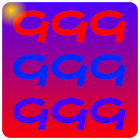999 Multiplication Table

All Android applications categories

All Android games categories# 999 Multiplication Table

by: 15 7.6

7.6 Users
rating

## Screenshots

Description

In mathematics, a multiplication table is a mathematical table used to define a multiplication operation for an algebraic system.

The decimal multiplication table was traditionally taught as an essential part of elementary arithmetic around the world, as it lays the foundation for arithmetic operations with our base-ten numbers. Many educators believe it is necessary to memorize the table up to 99 × 9.

Tags: multiplication table up to 100 , multiplication chart up to 100 , multiplication table to 100 , multiplication table upto 100 , multiplication chart to 100 , multiplication chart up to 10000 , multiplication chart , multiplication chart up to 1000 , multiplication tables up to 100

from 15 reviews

"Great"

7.6